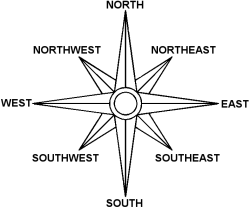### Problem Statement

The great pirate Silver has buried a treasure on a tropical island. You managed to find a map of the island with an X marking a spot on the island, and a set of instructions. Each instruction is of the form "walk A steps in the direction B". If you start at the X and follow the instructions in the given order, at the end you will arrive at the location where the treasure is buried.

Your evil twin has a copy of the map, and has already started to follow the instructions. To beat him, you must find the location of the treasure and walk straight to that place.

You will be given the set of instructions as a int[] steps and a String[] directions. Element i of directions corresponds to element i of steps. Write a method that will find the location of the treasure, and return the straight-line distance (in steps) from the place where you start to the place where the treasure is buried.

Each direction in directions will be one of the eight basic directions on a compass. Refer to the image below for clarification.### Definition

 Class: PirateTreasure Method: getDistance Parameters: int[], String[] Returns: double Method signature: double getDistance(int[] steps, String[] directions) (be sure your method is public)

### Notes

-The returned value must have an absolute or relative error less than 1e-9.
-Assume that the island lies on a plane, and that it is large enough to accomodate the entire walk.
-Note that making a step northwest is not the same as making a step north and then a step west. See Example 3.
-The Euclidean distance between two points A=(ax,ay) and B=(bx,by) in a plane is equal to sqrt( (ax-bx)^2 + (ay-by)^2 ).

### Constraints

-steps will contain between 1 and 50 elements, inclusive.
-Each element in steps will be between 1 and 50, inclusive.
-directions will contain the same number of elements as steps.
-Each element of directions will be one of NORTH, SOUTH, EAST, WEST, NORTHEAST, NORTHWEST, SOUTHEAST, and SOUTHWEST.

### Examples

0)

 `{1}` `{"NORTH"}`
`Returns: 1.0`
 After one step north, you will be (surprisingly) one step away from where you started.
1)

 `{2}` `{"NORTHWEST"}`
`Returns: 2.0`
 Two steps northwest will take you two steps away from where you started.
2)

 `{3,10,7}` `{"EAST","WEST","EAST"}`
`Returns: 0.0`
 After following these instructions you'll end exactly where you started.
3)

 `{34,48,34}` `{"NORTH","SOUTHWEST","EAST"}`
`Returns: 0.08326112068522587`
 Following these instructions won't get you exactly to the same place where you started, but you will be pretty close.
4)

 `{2,2,2,1}` `{"NORTH","NORTH","NORTH","NORTHWEST"}`
`Returns: 6.744277676240694`
 After six steps north and one step northwest, your straight line distance from where you started is a little less than seven steps.

#### Problem url:

http://www.topcoder.com/stat?c=problem_statement&pm=8241

#### Problem stats url:

http://www.topcoder.com/tc?module=ProblemDetail&rd=10936&pm=8241

misof

#### Testers:

PabloGilberto , vorthys , Olexiy , ivan_metelsky

#### Problem categories:

Geometry, Simulation